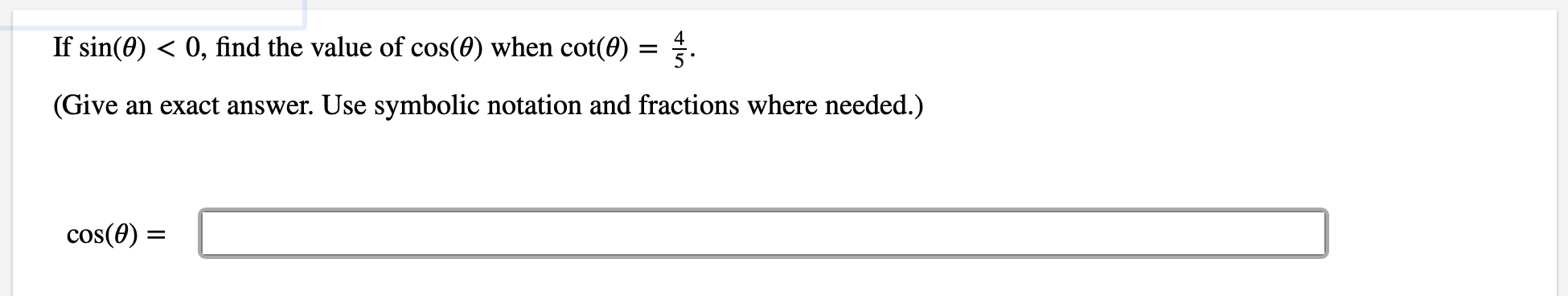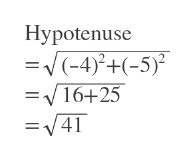0, find the value of cos(0) when cot(0) = 4.If sin(0)(Givean exact answer. Use symbolic notation and fractions where needed.)cos(0)

Questionhelp_outlineImage Transcriptionclose0, find the value of cos(0) when cot(0) = 4. If sin(0) (Give an exact answer. Use symbolic notation and fractions where needed.) cos(0) fullscreen
Step 1

sin theta<0 but cot theta>0

So this must be 3rd quadrant.

There cos theta will be negative

Step 2

First we draw a right tirnagle in 3rd quadrant so that opposite = -5 and adjacent = -4

Step 3

Then we find hypotenuse of it.

...help_outlineImage TranscriptioncloseHypotenuse 2 (-4) =16+25 =V41 +(-5 II fullscreen

Want to see the full answer?

See Solution

Want to see this answer and more?

Our solutions are written by experts, many with advanced degrees, and available 24/7

See Solution
Tagged in

Other